# The Remainder Formula Excel: Everything You Need to Know

Mar 14, 2023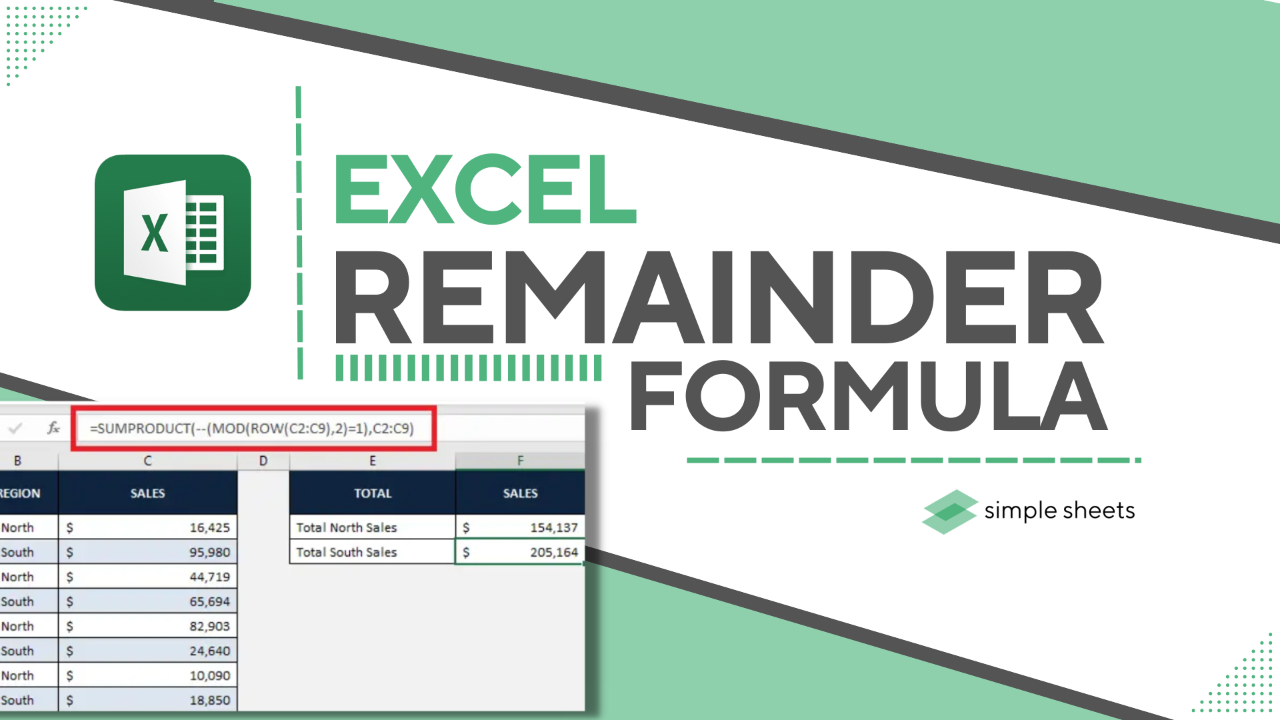Are you looking for a way to calculate your remainder in Microsoft Excel?

We've got you covered with our step-by-step instructions. Even beginners can quickly learn how to calculate remainders like a pro using the built-in MOD function within Excel.

Read on as we cover the following:

• How to calculate the remainder using the MOD function.

• Final thoughts on using the remainder formula in Excel.

• Frequently asked questions on the remainder formula Excel.## Calculate the Remainder Using the MOD Function

To get the remainder, you must use the MOD Function in Excel. This function calculates the remainder after a division operation.

### MOD Function Syntax.

=MOD(Value to be divided, Value to divide by)

### How to calculate the remainder.

1. In the remainder cell, type the following formula of the MOD function in the image, click row number (12,3), and press enter key in your Excel spreadsheet.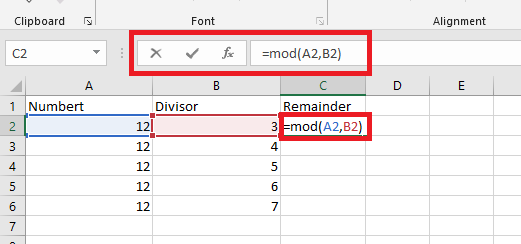1. Use the drag-fill option to calculate cells for the remainder of the following rows.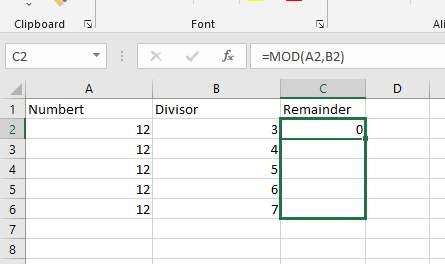Note:

1. The outcome of the MOD function has the same sign as the divisor.

2. If you try to divide by zero, the MOD function returns 0.

3. Excel will give an error value if you use words instead of numbers in a division problem.

### Add up every other row.

To add up the numbers in every second row, use MOD with ROW and SUMPRODUCT.

This will give you the sum of all the even rows:

=SUMPRODUCT((MOD(ROW(range),2)=0)*(range))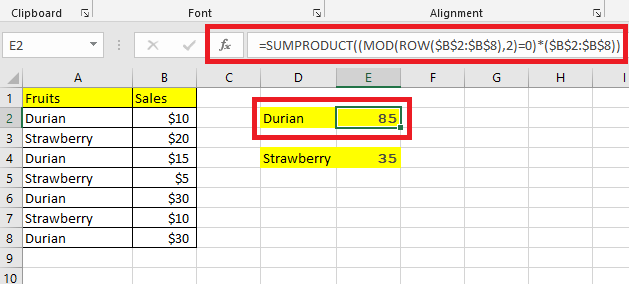This will provide you with the sum of all the odd rows:

=SUMPRODUCT((MOD(ROW(range),2)=1)*(range))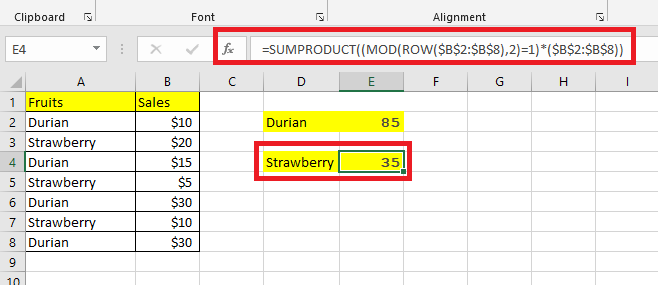You can use the IF function and SUM to do the same thing as using SUMPRODUCT. The IF function checks if something is true or false, and SUM adds up numbers (it's an array formula typed by pressing Ctrl + Shift + Enter).

=SUM(IF(MOD(ROW(\$B\$2:\$B\$8),2)=0,\$B\$2:\$B\$8,0))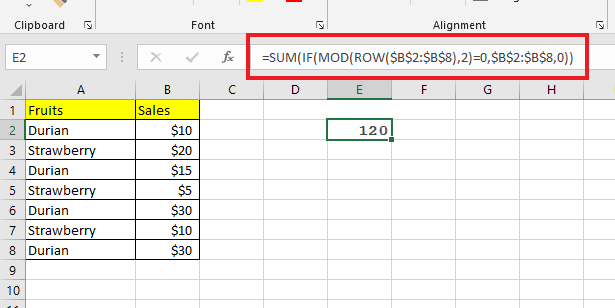## Final Thoughts on the Remainder Formula Excel

Sometimes using the remainder formula can lead to mistakes, so checking your answer twice is essential. Knowing how to use the Excel MOD function correctly can help you with data analysis tasks that need you to calculate remainders.## Frequently Asked Questions on the Remainder Formula Excel

### Should I use Conditional Formatting when getting the remainder using the MOD Function?

Applying Conditional Formatting will display your cells having remainder on it, which can help you in data visualization.

### Can I concatenate every N cell?

Yes, Your formula will be able to work with any data, no matter where it is in the row. It won't need to be changed again.

### How do I validate my remainder formula with Data Validation option?

1. Go to the Data tab.

2. Choose Data Validation.

3. On the Settings tab, select Custom in the Allow box.

4. Enter your formula in the Formula box.## Related Articles

Excel Remainder: How to Get it With the Excel MOD Function

How to Use SUMPRODUCT with Multiple Criteria in Excel

The SUMIFS Google Sheets Function: Everything You Need To Know

#### Want to Make Excel Work for You? Try out 5 Amazing Excel Templates & 5 Unique Lessons

We hate SPAM. We will never sell your information, for any reason.